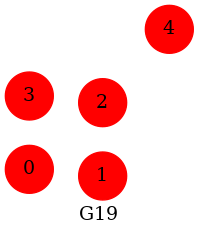# Atlas¶

An example showing how to write first 20 graphs from the graph atlas as graphviz dot files Gn.dot where n=0,19.

TODO: does nx_agraph.draw support multiple graphs in one png?Out:

```Graph named 'G0' with 0 nodes and 0 edges
Graph named 'G1' with 1 nodes and 0 edges
Graph named 'G2' with 2 nodes and 0 edges
Graph named 'G3' with 2 nodes and 1 edges
Graph named 'G4' with 3 nodes and 0 edges
Graph named 'G5' with 3 nodes and 1 edges
Graph named 'G6' with 3 nodes and 2 edges
Graph named 'G7' with 3 nodes and 3 edges
Graph named 'G8' with 4 nodes and 0 edges
Graph named 'G9' with 4 nodes and 1 edges
Graph named 'G10' with 4 nodes and 2 edges
Graph named 'G11' with 4 nodes and 2 edges
Graph named 'G12' with 4 nodes and 3 edges
Graph named 'G13' with 4 nodes and 3 edges
Graph named 'G14' with 4 nodes and 3 edges
Graph named 'G15' with 4 nodes and 4 edges
Graph named 'G16' with 4 nodes and 4 edges
Graph named 'G17' with 4 nodes and 5 edges
Graph named 'G18' with 4 nodes and 6 edges
Graph named 'G19' with 5 nodes and 0 edges
```

```import networkx as nx

atlas = nx.graph_atlas_g()[0:20]

for G in atlas:
print(G)
A = nx.nx_agraph.to_agraph(G)
A.graph_attr["label"] = G.name
# set default node attributes
A.node_attr["color"] = "red"
A.node_attr["style"] = "filled"
A.node_attr["shape"] = "circle"
A.write(G.name + ".dot")

# Draw the 20th graph from the atlas to png
A.draw("A20.png", prog="neato")
```

Total running time of the script: ( 0 minutes 0.088 seconds)

Gallery generated by Sphinx-Gallery